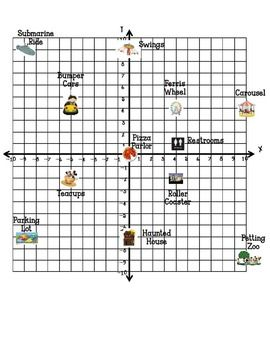Kindergarten
1st
2nd
3rd
4th
5th
6th
7th
8th
9th
10th
11th
12th
Higher Ed
Other
Subjects
ELA
Math
Science
Social Studies
Art
Computer Science
French
German
Music
Physical Education
Spanish
Other
Private Library
Math Practice Test #1
starstarstarstarstarstarstarstarstarstar
by Angela JOhnson
| 6 Questions
Note from the author:
MS CC Standards OA, G, NF, NBT
1
1 pt
1. The football team has a 25-gallon cooler filled with water for each practice. There are 35 players on the team. Which fraction represents the amount of water, in gallons, each player receives if the water is shared equally among them?
A 35/25 gallons
B 25/35 gallons
C 29/25 gallons
D 7/5 gallons
2
2 pts
Directions: Evaluate the expression 6 + 30 ÷ 3 × (5 − 2)
A 58
B 48
C 36
D 14
3
2 pts
Which statement describes the relationship between the value of 4 in the number 5,347,129 and the value of 4 in the number 4,823,165?
A. In the number 5,347,129 , the value of 4 is 1/10 the value of 4 in the number 4,823,165
B. In the number 5,347,129 , the value of 4 is 1/100 the value of 4 in the number 4,823,165
C. In the number 5,347,129 , the value of 4 is 10times the value of 4 in the number 4,823,165
D. In the number 5,347,129 , the value of 4 is100 times the value of 4 in the number 4,823,165
4
3 pts
Directions: Select all the ways that you can rewrite the decimal 2.84
A 284 ones
B 2 + 8/10 + 4/100
C 2(1) + 8(0.1) + 4(0.01)
D Two and eighty-four hundredths
E Two and eighty-four tenths
5
1 pt
Which statement below best explains how to evaluate the expression 14/15 - 2/3
A Subtract the numerators first then subtract the denominators.
B Write an equivalent fraction for 14/15 with 3 as the new numerator. Then subtract the numerators and the denominators separately.
C Write equivalent fractions for both fractions where 45 is the denominator. Then subtract the numerators and the denominators separately.
D Write an equivalent fraction for 2/3 with 15 as the new denominator. Then subtract only the numerators6
1 pt
Use the graph above to answer the question.
Which point has the ordered pair (4,1) ?
A Parking lot
B Haunted house
C Roller coaster
D Restrooms
Add to my formatives list